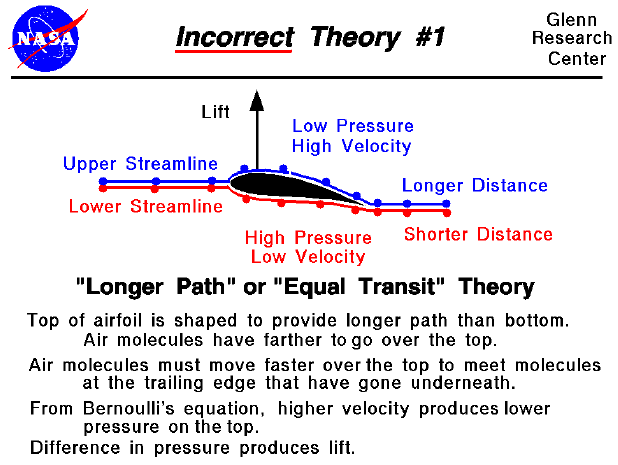There are many theories of how lift is generated. Unfortunately, many of the theories found in encyclopedias, on web sites, and even in some textbooks are incorrect, causing unnecessary confusion for students.

The theory described on this slide is one of the most widely circulated, incorrect explanations. The theory can be labeled the "Longer Path" theory, or the "Equal Transit Time" theory. The theory states that airfoils are shaped with the upper surface longer than the bottom. The air molecules (the little colored balls on the figure) have farther to travel over the top of the airfoil than along the bottom in order to meet up at the trailing edge. Therefore, the molecules going over the top of the wing must travel faster than the molecules moving under the wing. Because the upper flow is faster, then, from Bernoulli's equation, the pressure is lower. The difference in pressure across the airfoil produces the lift.

Before considering what is wrong with this theory, let's investigate the actual flow around an airfoil by doing a couple of experiments using a Java simulator which is solving the correct flow equations.

This interactive Java applet shows flow going past a symmetric airfoil. The flow is shown by a series of moving particles. You can change the angle of attack of the airfoil by using a slider, and the angle of attack generates the lift through flow turning. There is also a translating probe with a gage on the simulator which lets you investigate the flow.

This is a secondary Java applet which uses a text box to describe some experiments for the student to perform using the previous applet.

Let's use the information we've just learned to evaluate the various parts of the "Equal Transit" Theory.

• {Lifting airfoils are designed to have the upper surface longer than the bottom.} This is not always correct. The symmetric airfoil in our experiment generates plenty of lift and its upper surface is the same length as the lower surface. Think of a paper airplane. Its airfoil is a flat plate --> top and bottom exactly the same length and shape and yet they fly just fine. This part of the theory probably got started because early airfoils were curved and shaped with a longer distance along the top. Such airfoils do produce a lot of lift and flow turning, but it is the turning that's important, not the distance. There are modern, low-drag airfoils which produce lift on which the bottom surface is actually longer than the top. This theory also does not explain how airplanes can fly upside-down (the longer path would then be on the bottom!) which happens often at air shows and in air-to-air combat.
• {Air molecules travel faster over the top to meet molecules moving underneath at the trailing edge.} Experiment #1 shows us that the flow over the top of a lifting airfoil does travel faster than the flow beneath the airfoil. But the flow is much faster than the speed required to have the molecules meet up at the trailing edge. Two molecules near each other at the leading edge will not end up next to each other at the trailing edge as shown in Experiment #2. This part of the theory attempts to provide us with a value for the velocity over the top of the airfoil based on a non-physical assumption (the molecules meet at the aft end). We can calculate a velocity based on this assumption, and use Bernoulli's equation to compute the pressure, and perform the pressure-area calculation and the answer we get does not agree with the lift that we measure for a given airfoil. The lift predicted by the "Equal Transit" theory is much less than the observed lift, because the velocity is too low. The actual velocity over the top of an airfoil is much faster than that predicted by the "Longer Path" theory and particles moving over the top arrive at the trailing edge before particles moving under the airfoil.

• {The upper flow is faster and from Bernoulli's equation the pressure is lower. The difference in pressure across the airfoil produces the lift.} As we have seen in Experiment #1, this part of the theory is correct. In fact, this theory is very appealing because many parts of the theory are correct. In our discussions on pressure-area integration to determine the force on a body immersed in a fluid, we mentioned that if we know the velocity, we can obtain the pressure and determine the force. The problem with the "Equal Transit" theory is that it attempts to provide us with the velocity based on a non-physical assumption as discussed above.

You can further investigate all the factors affecting lift by using the FoilSim II Java Applet. You can also download your own copy of FoilSim to play with for free.Guided Tours